Visualizations

Databricks supports various types of visualizations out of the box using the display and displayHTML functions.

Databricks also natively supports visualization libraries in Python and R and lets you install and use third-party libraries.

display function

The display function supports several data and visualization types.

Data types

DataFrames

The easiest way to create a DataFrame visualization in Databricks is to call display(<dataframe-name>). For example, if you have a Spark DataFrame diamonds_df of a diamonds dataset grouped by diamond color, computing the average price, and you call:

from pyspark.sql.functions import avg

display(diamonds_df.select("color","price").groupBy("color").agg(avg("price")))

A table of diamond color versus average price appears.Tip

If you see OK with no rendering after calling the display function, mostly likely the DataFrame or collection you passed in is empty.

display() supports pandas DataFrames. If you reference a DataFrame in pandas or Pandas API on Spark without display, the table is rendered as it would be in a Jupyter notebook.

DataFrame display method

Note

Available in Databricks Runtime 7.1 and above.

DataFrames in PySpark, pandas, and Pandas API on Spark have a display method that calls the Databricks display function. You can call it after a simple DataFrame operation, for example:

diamonds_df.select("color","price").display()

or at the end of a series of chained DataFrame operations, for example:

from pyspark.sql.functions import avg

diamonds_df.select("color","price").groupBy("color").agg(avg("price")).display()
Data profiles

Preview

This feature is in Public Preview.

Note

Available in Databricks Runtime 9.1 and above.

Starting with Databricks Runtime 9.1, when you use the DataFrame display method, two tabs appear in the results pane: Table and Data Profile. Table shows the results in tabular format and gives you access to built-in Plot types. Data Profile displays summary statistics of the Apache Spark or pandas DataFrame in tabular and graphic format.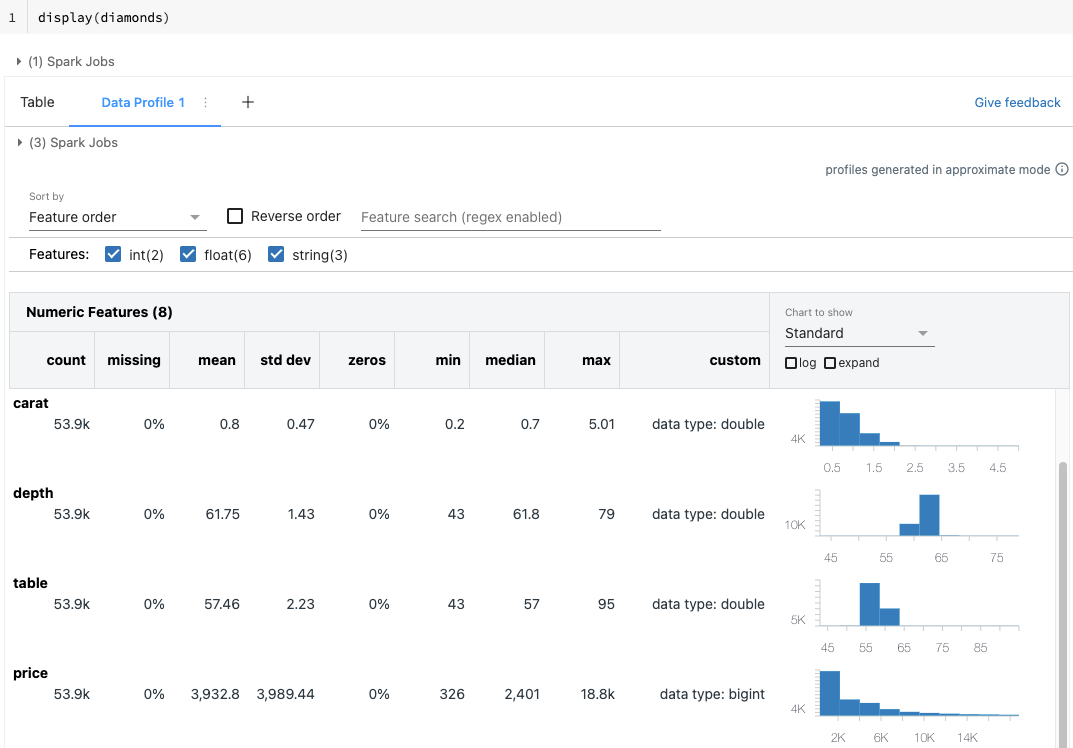• Numeric and categorical features are shown in separate tables.
• At the top of the tab, you can sort or search for features.
• At the top of the chart column, you can choose to display a histogram (Standard) or quantiles.
• Check expand to enlarge the charts.
• Check log to display the charts on a log scale.
• You can hover your cursor over the charts for more detailed information, such as the boundaries of a histogram column and the number of rows in it, or the quantile value.

Note

To see data profiles, you must use the syntax display(df). The syntax df.display() is not supported.

You can also generate data profiles programmatically; see summarize command (dbutils.data.summarize).

Images

display renders columns containing image data types as rich HTML. display attempts to render image thumbnails for DataFrame columns matching the Spark ImageSchema. Thumbnail rendering works for any images successfully read in through the spark.read.format('image') function. For image values generated through other means, Databricks supports the rendering of 1, 3, or 4 channel images (where each channel consists of a single byte), with the following constraints:

• One-channel images: mode field must be equal to 0. height, width, and nChannels fields must accurately describe the binary image data in the data field
• Three-channel images: mode field must be equal to 16. height, width, and nChannels fields must accurately describe the binary image data in the data field. The data field must contain pixel data in three-byte chunks, with the channel ordering (blue, green, red) for each pixel.
• Four-channel images: mode field must be equal to 24. height, width, and nChannels fields must accurately describe the binary image data in the data field. The data field must contain pixel data in four-byte chunks, with the channel ordering (blue, green, red, alpha) for each pixel.
Example

Suppose you have a folder containing some images: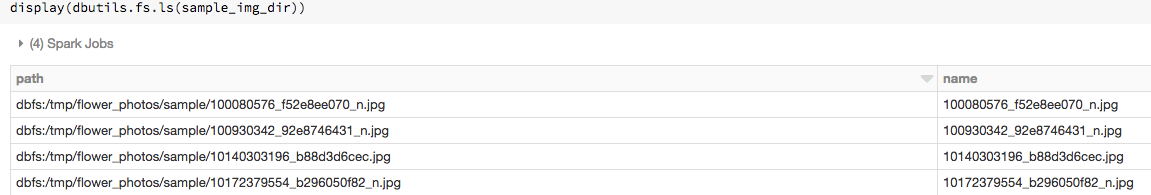If you read the images into a DataFrame with ImageSchema.readImages and then display the DataFrame, display renders thumbnails of the images:

from pyspark.ml.image import ImageSchema
display(image_df)Structured Streaming DataFrames

To visualize the result of a streaming query in real time you can display a Structured Streaming DataFrame in Scala and Python.

display(streaming_df.groupBy().count())
display(streaming_df.groupBy().count())

display supports the following optional parameters:

• streamName: the streaming query name.
• trigger (Scala) and processingTime (Python): defines how often the streaming query is run. If not specified, the system checks for availability of new data as soon as the previous processing has completed. To reduce the cost in production, Databricks recommends that you always set a trigger interval. With Databricks Runtime 8.0 and above, the default trigger interval is 500 ms.
• checkpointLocation: the location where the system writes all the checkpoint information. If it is not specified, the system automatically generates a temporary checkpoint location on DBFS. In order for your stream to continue processing data from where it left off, you must provide a checkpoint location. Databricks recommends that in production you always specify the checkpointLocation option.
display(streaming_df.groupBy().count(), processingTime = "5 seconds", checkpointLocation = "dbfs:/<checkpoint-path>")
import org.apache.spark.sql.streaming.Trigger

display(streaming_df.groupBy().count(), trigger = Trigger.ProcessingTime("5 seconds"), checkpointLocation = "dbfs:/<checkpoint-path>")

Plot types

The display function supports a rich set of plot types: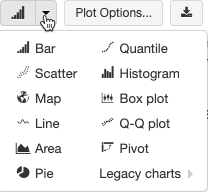Choose and configure a chart type

To choose a bar chart, click the bar chart icon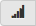: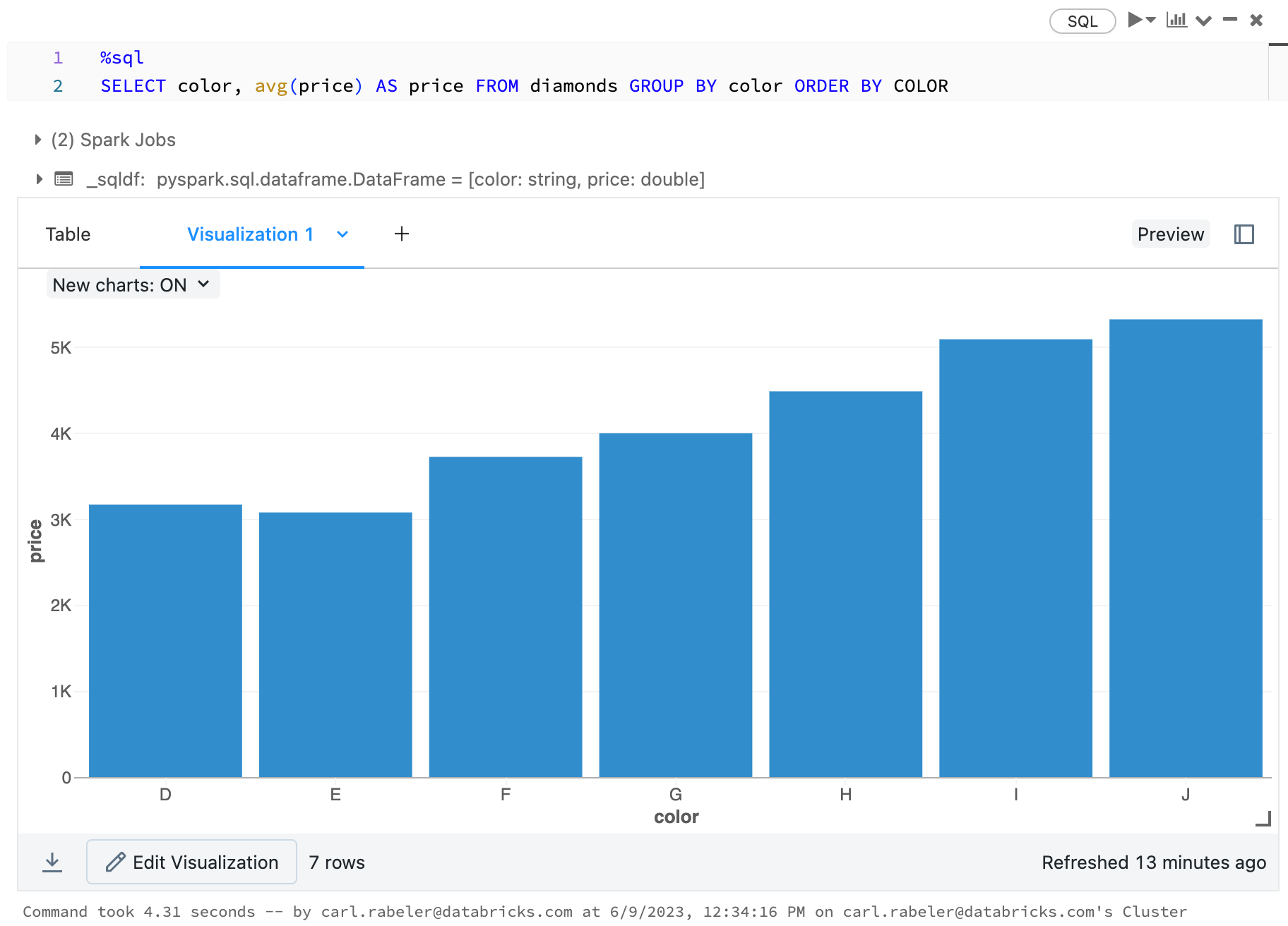To choose another plot type, clickto the right of the bar chartand choose the plot type.

Chart toolbar

Both line and bar charts have a built-in toolbar that support a rich set of client-side interactions.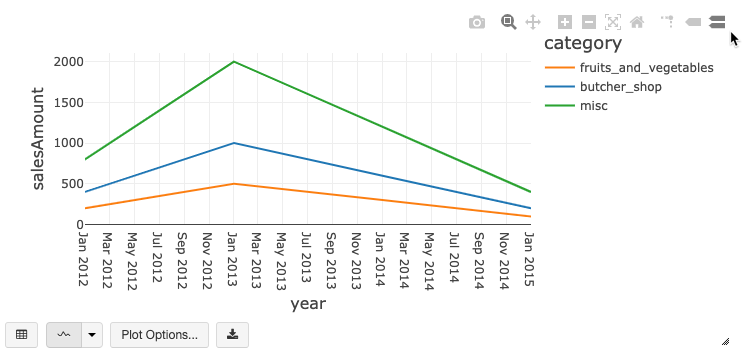To configure a chart, click Plot Options….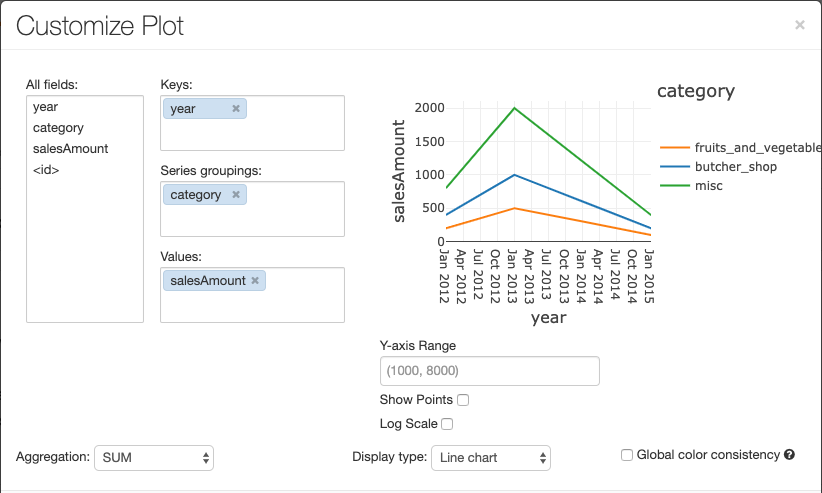The line chart has a few custom chart options: setting a Y-axis range, showing and hiding points, and displaying the Y-axis with a log scale.

For information about legacy chart types, see:

Color consistency across charts

Databricks supports two kinds of color consistency across charts: series set and global.

Series set color consistency assigns the same color to the same value if you have series with the same values but in different orders (for example, A = ["Apple", "Orange", "Banana"] and B = ["Orange", "Banana", "Apple"]). The values are sorted before plotting, so both legends are sorted the same way (["Apple", "Banana", "Orange"]), and the same values are given the same colors. However, if you have a series C = ["Orange", "Banana"], it would not be color consistent with set A because the set isn’t the same. The sorting algorithm would assign the first color to “Banana” in set C but the second color to “Banana” in set A. If you want these series to be color consistent, you can specify that charts should have global color consistency.

In global color consistency, each value is always mapped to the same color no matter what values the series have. To enable this for each chart, select the Global color consistency checkbox.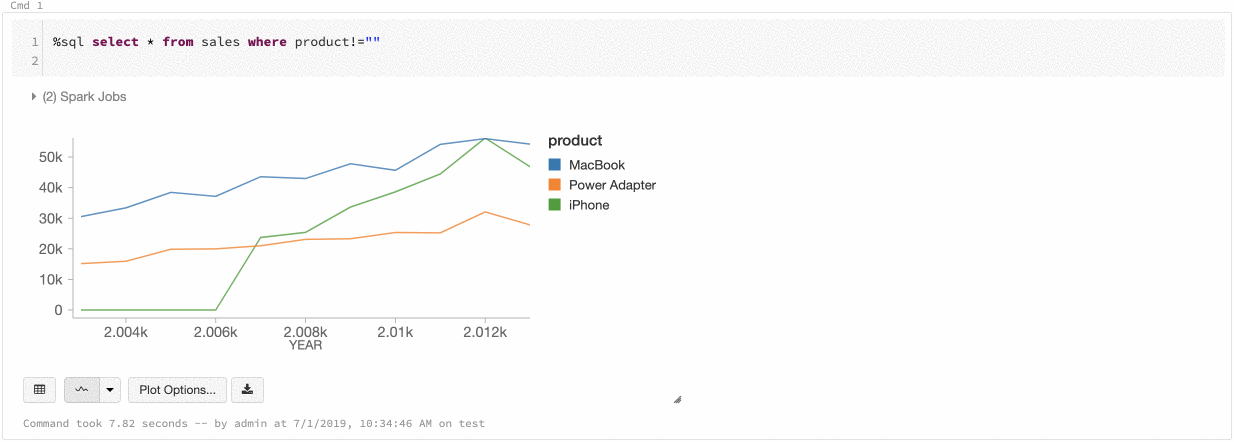Note

To do achieve this consistency, Databricks hashes directly from values to colors. To avoid collisions (where two values go to the exact same color), the hash is to a large set of colors, which has the side effect that nice-looking or easily distinguishable colors cannot be guaranteed; with many colors there are bound to be some that are very similar looking.

Machine learning visualizations

In addition to the standard chart types, the display function supports visualizations of the following machine learning training parameters and results:

Residuals

For linear and logistic regressions, display supports rendering a fitted versus residuals plot. To obtain this plot, you supply the model and DataFrame.

The following example runs a linear regression on city population to house sale price data and then displays the residuals versus the fitted data.

# Drop rows with missing values and rename the feature and label columns, replacing spaces with _
from pyspark.sql.functions import col
pop_df = pop_df.dropna() # drop rows with missing values
exprs = [col(column).alias(column.replace(' ', '_')) for column in pop_df.columns]

# Register a UDF to convert the feature (2014_Population_estimate) column vector to a VectorUDT type and apply it to the column.
from pyspark.ml.linalg import Vectors, VectorUDT

spark.udf.register("oneElementVec", lambda d: Vectors.dense([d]), returnType=VectorUDT())
tdata = pop_df.select(*exprs).selectExpr("oneElementVec(2014_Population_estimate) as features", "2015_median_sales_price as label")

# Run a linear regression
from pyspark.ml.regression import LinearRegression

lr = LinearRegression()
modelA = lr.fit(tdata, {lr.regParam:0.0})

# Plot residuals versus fitted data
display(modelA, tdata)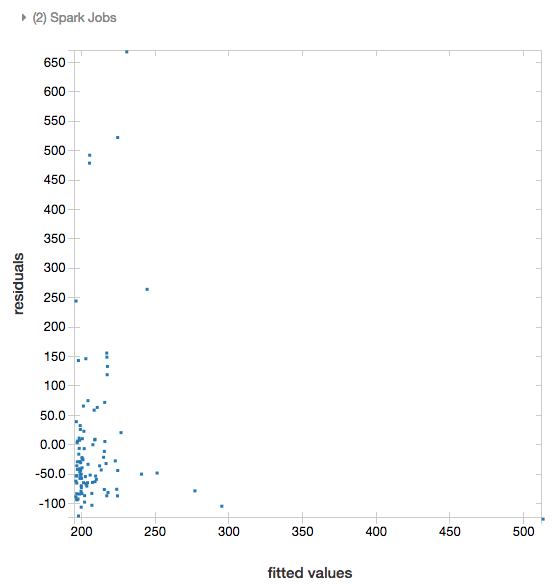ROC curves

For logistic regressions, display supports rendering an ROC curve. To obtain this plot, you supply the model, the prepped data that is input to the fit method, and the parameter "ROC".

The following example develops a classifier that predicts if an individual earns <=50K or >50k a year from various attributes of the individual. The Adult dataset derives from census data, and consists of information about 48842 individuals and their annual income.

The example code in this section uses one-hot encoding. The function was renamed with Apache Spark 3.0, so the code is slightly different depending on the version of Databricks Runtime you are using. If you are using Databricks Runtime 6.x or below, you must adjust two lines in the code as described in the code comments.

# This code uses one-hot encoding to convert all categorical variables into binary vectors.

schema = """`age` DOUBLE,
`workclass` STRING,
`fnlwgt` DOUBLE,
`education` STRING,
`education_num` DOUBLE,
`marital_status` STRING,
`occupation` STRING,
`relationship` STRING,
`race` STRING,
`sex` STRING,
`capital_gain` DOUBLE,
`capital_loss` DOUBLE,
`hours_per_week` DOUBLE,
`native_country` STRING,
`income` STRING"""

from pyspark.ml import Pipeline
from pyspark.ml.feature import OneHotEncoder, StringIndexer, VectorAssembler
# If you are using Databricks Runtime 6.x or below, comment out the preceding line and uncomment the following line.
# from pyspark.ml.feature import OneHotEncoderEstimator, StringIndexer, VectorAssembler
categoricalColumns = ["workclass", "education", "marital_status", "occupation", "relationship", "race", "sex", "native_country"]

stages = [] # stages in the Pipeline
for categoricalCol in categoricalColumns:
# Category indexing with StringIndexer
stringIndexer = StringIndexer(inputCol=categoricalCol, outputCol=categoricalCol + "Index")
# Use OneHotEncoder to convert categorical variables into binary SparseVectors
encoder = OneHotEncoder(inputCols=[stringIndexer.getOutputCol()], outputCols=[categoricalCol + "classVec"])
# If you are using Databricks Runtime 6.x or below, comment out the preceding line and uncomment the following line.
# encoder = OneHotEncoderEstimator(inputCols=[stringIndexer.getOutputCol()], outputCols=[categoricalCol + "classVec"])
# Add stages.  These are not run here, but will run all at once later on.
stages += [stringIndexer, encoder]

# Convert label into label indices using the StringIndexer
label_stringIdx = StringIndexer(inputCol="income", outputCol="label")
stages += [label_stringIdx]

# Transform all features into a vector using VectorAssembler
numericCols = ["age", "fnlwgt", "education_num", "capital_gain", "capital_loss", "hours_per_week"]
assemblerInputs = [c + "classVec" for c in categoricalColumns] + numericCols
assembler = VectorAssembler(inputCols=assemblerInputs, outputCol="features")
stages += [assembler]

# Run the stages as a Pipeline. This puts the data through all of the feature transformations in a single call.

partialPipeline = Pipeline().setStages(stages)
pipelineModel = partialPipeline.fit(dataset)

# Fit logistic regression model

from pyspark.ml.classification import LogisticRegression

# ROC for dataTo display the residuals, omit the "ROC" parameter:Decision trees

The display function supports rendering a decision tree.

To obtain this visualization, you supply the decision tree model.

The following examples train a tree to recognize digits (0 - 9) from the MNIST dataset of images of handwritten digits and then displays the tree.

from pyspark.ml.classification import DecisionTreeClassifier
from pyspark.ml.feature import StringIndexer
from pyspark.ml import Pipeline

indexer = StringIndexer().setInputCol("label").setOutputCol("indexedLabel")

dtc = DecisionTreeClassifier().setLabelCol("indexedLabel")

# Chain indexer + dtc together into a single ML Pipeline.
pipeline = Pipeline().setStages([indexer, dtc])

model = pipeline.fit(trainingDF)
display(model.stages[-1])

import org.apache.spark.ml.classification.{DecisionTreeClassifier, DecisionTreeClassificationModel}
import org.apache.spark.ml.feature.StringIndexer
import org.apache.spark.ml.Pipeline

val indexer = new StringIndexer().setInputCol("label").setOutputCol("indexedLabel")
val dtc = new DecisionTreeClassifier().setLabelCol("indexedLabel")
val pipeline = new Pipeline().setStages(Array(indexer, dtc))

val model = pipeline.fit(trainingDF)
val tree = model.stages.last.asInstanceOf[DecisionTreeClassificationModel]

display(tree)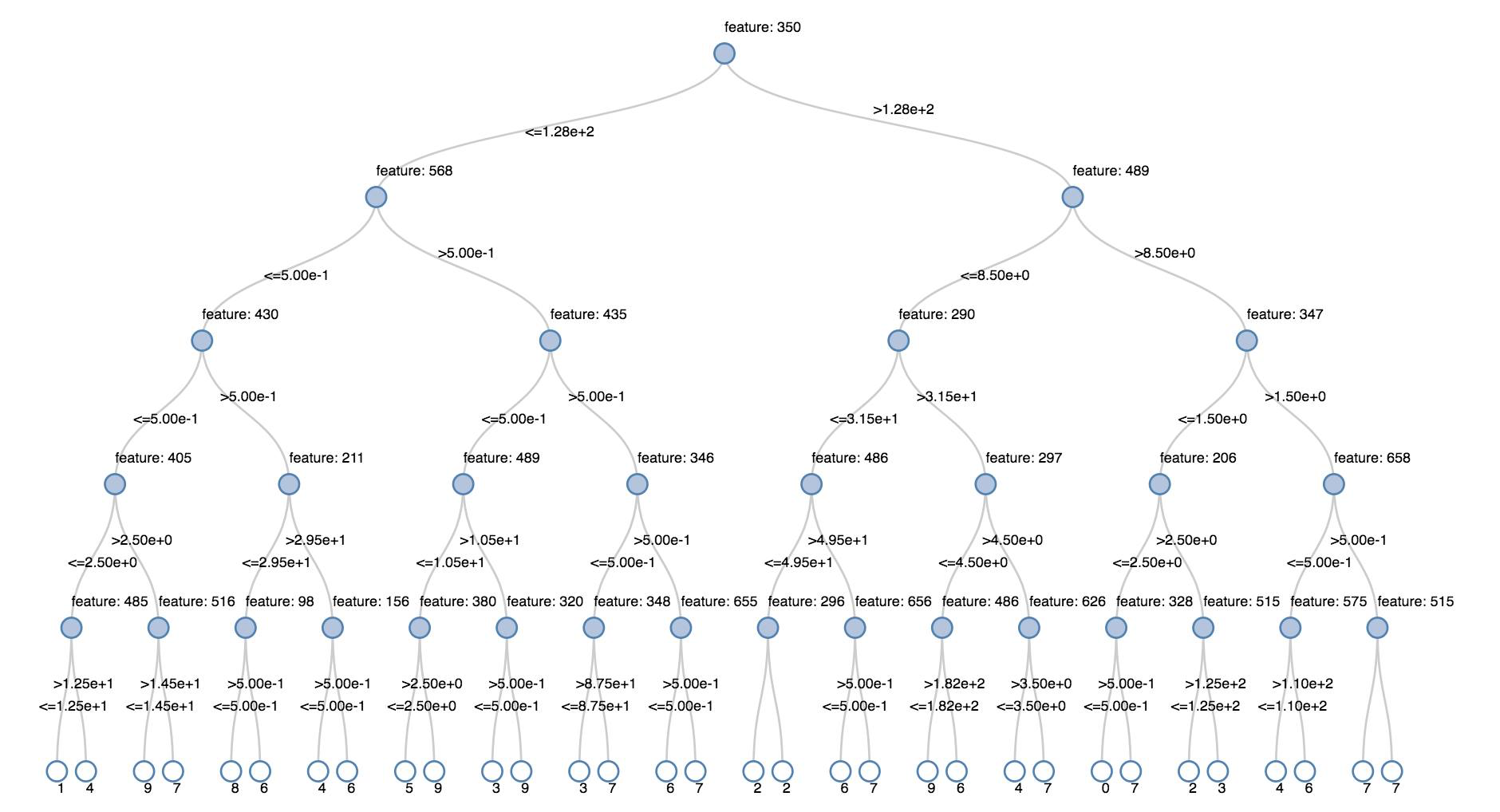displayHTML function

Databricks programming language notebooks (Python, R, and Scala) support HTML graphics using the displayHTML function; you can pass the function any HTML, CSS, or JavaScript code. This function supports interactive graphics using JavaScript libraries such as D3.

For examples of using displayHTML, see:

Note

The displayHTML iframe is served from the domain databricksusercontent.com, and the iframe sandbox includes the allow-same-origin attribute. databricksusercontent.com must be accessible from your browser. If it is currently blocked by your corporate network, it must added to an allow list.

Visualizations by language

Visualizations in Python

To plot data in Python, use the display function as follows:

display(diamonds_df.groupBy("color").avg("price").orderBy("color"))In this section:

Deep dive Python notebook

For a deep dive into Python visualizations using display, see the notebook:

Seaborn

You can also use other Python libraries to generate plots. The Databricks Runtime includes the seaborn visualization library. To create a seaborn plot, import the library, create a plot, and pass the plot to the display function.

import seaborn as sns
sns.set(style="white")

g = sns.PairGrid(df, diag_sharey=False)
g.map_lower(sns.kdeplot)
g.map_diag(sns.kdeplot, lw=3)

g.map_upper(sns.regplot)

display(g.fig)Visualizations in R

To plot data in R, use the display function as follows:

library(SparkR)

display(arrange(agg(groupBy(diamonds_df, "color"), "price" = "avg"), "color"))

You can use the default R plot function.

fit <- lm(Petal.Length ~., data = iris)
layout(matrix(c(1,2,3,4),2,2)) # optional 4 graphs/page
plot(fit)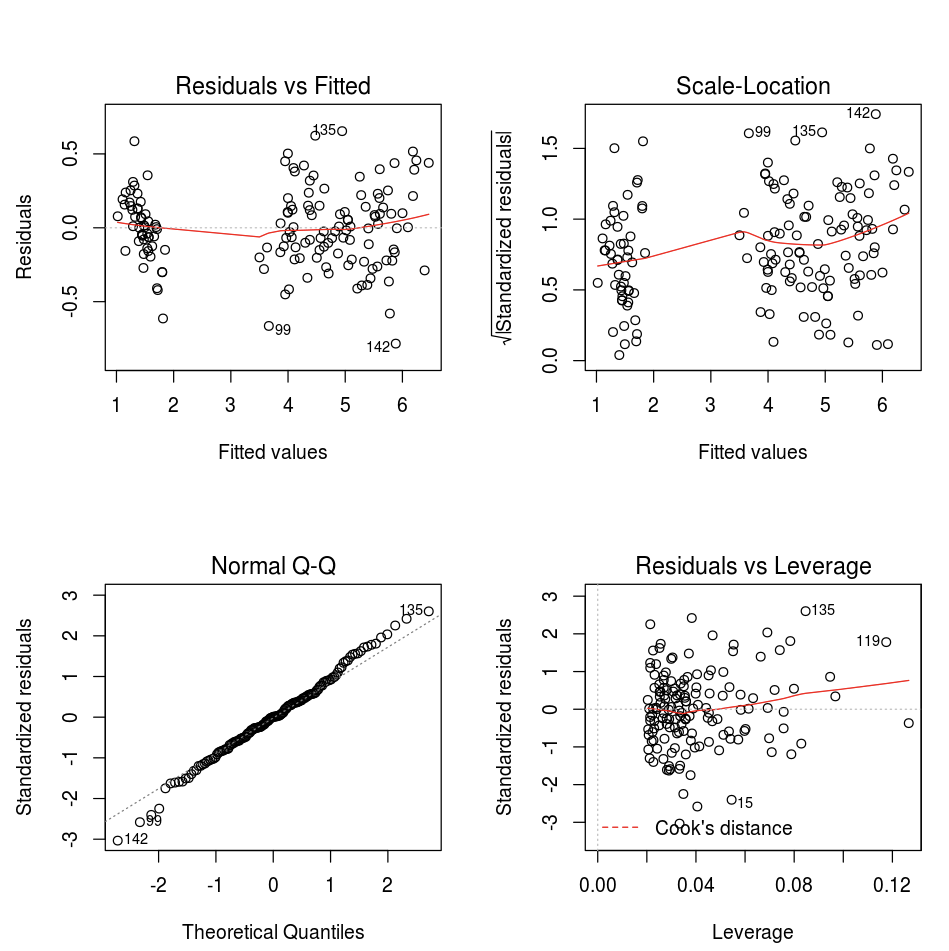You can also use any R visualization package. The R notebook captures the resulting plot as a .png and displays it inline.

In this section:

Lattice

The Lattice package supports trellis graphs—graphs that display a variable or the relationship between variables, conditioned on one or more other variables.

library(lattice)
xyplot(price ~ carat | cut, diamonds, scales = list(log = TRUE), type = c("p", "g", "smooth"), ylab = "Log price")DandEFA

The DandEFA package supports dandelion plots.

install.packages("DandEFA", repos = "https://cran.us.r-project.org")
library(DandEFA)
data(timss2011)
timss2011 <- na.omit(timss2011)
dandpal <- rev(rainbow(100, start = 0, end = 0.2))
dandelion(facl,bound=0,mcex=c(1,1.2),palet=dandpal)
dandelion(facl,bound=0,mcex=c(1,1.2),palet=dandpal)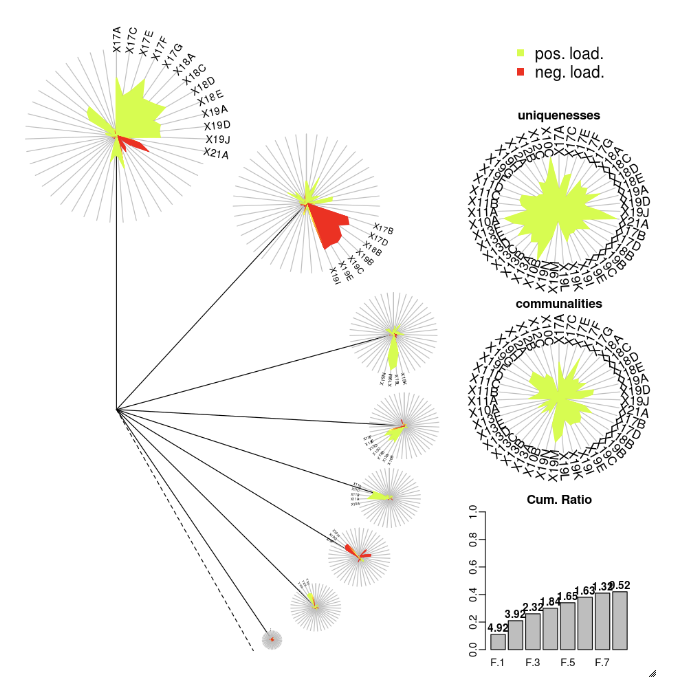Plotly

The Plotly R package relies on htmlwidgets for R. For installation instructions and a notebook, see htmlwidgets.

Visualizations in Scala

To plot data in Scala, use the display function as follows:

display(diamonds_df.groupBy("color").avg("price").orderBy("color"))Deep dive Scala notebook

For a deep dive into Scala visualizations using display, see the notebook:

Visualizations in SQL

When you run a SQL query, Databricks automatically extracts some of the data and displays it as a table.

SELECT color, avg(price) AS price FROM diamonds GROUP BY color ORDER BY COLORFrom there you can select different chart types.Data profiles in SQL

Preview

This feature is in Public Preview.

Starting with Databricks Runtime 9.1, two tabs appear in the results pane: Table and Data Profile. Table shows the results in tabular format and gives you access to the different chart types. Data Profile displays summary statistics of the table in tabular and graphic format. For an example display and details about the data profiles shown, see Data profiles.